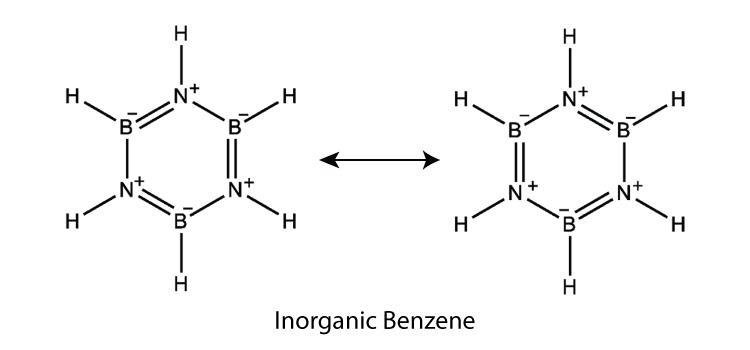# The formula of inorganic benzene is________

The formula of inorganic benzene is (BH)3(NH)3

## Formula of Inorganic Benzene

Inorganic benzene is also known as borazine which is an inorganic and a cyclic compound. There are three BH units and three NH units alternate. The chemical formula of inorganic benzene is:

 (BH)3(NH)3

### Inorganic Benzene Structure### Why is borazine called ‘inorganic benzene’?

Borazine is called ‘inorganic benzene’ because the compound is isoelectronic and isostructural with benzene. The other similarities are it is a colourless liquid with aromatic smell. Borazine is said to be aromatic because the number of pi electrons obeys 4n+2 rule and the B-N bond lengths are all equal.

### Preparation of Inorganic Benzene

There are three different ways to prepare inorganic benzene and they are:

• Stock and Pohlands method:

$$\begin{array}{l}3B_{2}H_{6}+6NH_{3}\rightarrow 3[B_{2}H_{6}.NH_{3}]\rightarrow 2B_{3}N_{3}H_{6}+12H_{2}\end{array}$$

The yield obtained by this method is low because there is a simultaneous formation of solid polymeric by-products.

• Heating BCl3 with NH4Cl:

$$\begin{array}{l}3NH_{4}Cl+3BCl_{3}\rightarrow[C_{6}H_{5}cl,Fe]{140^{\circ} C}Cl_{3}B_{3}N_{3}H_{3}+9HCl\end{array}$$

$$\begin{array}{l}3Cl_{3}B_{3}N_{3}H_{3}+6LiBH_{4}\overset{[H]}{\rightarrow}2B_{3}N_{3}H_{6}+6LiCl+3B_{2}H_{6}\end{array}$$

• By heating mixture of LiBH4 and NH4Cl:

$$\begin{array}{l}3NH_{4}Cl+3LiBH_{4}\overset{230^{\circ}C}{\rightarrow}B_{3}N_{3}H_{6}+3LiCl+9H_{2}\end{array}$$

This method gives up to 30% Borazine.(13)(0)